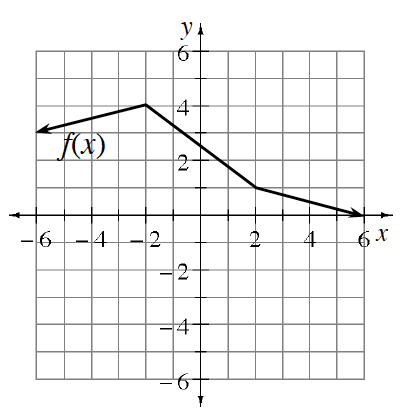### Home > INT1 > Chapter Ch11 > Lesson 11.2.3 > Problem11-87

11-87.

Given the graph of $f(x)$ right, graph each transformation listed below.

1. $f(x) − 5$

2. $f(x) + 1.5$